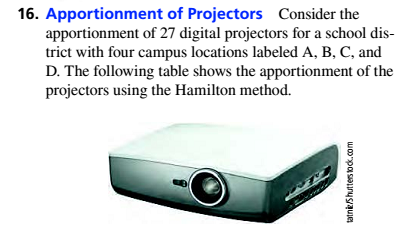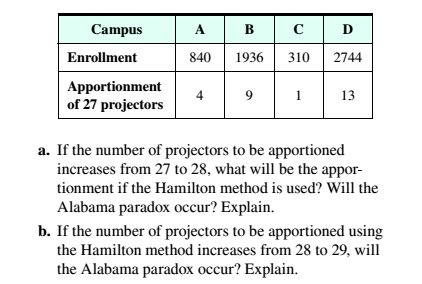# Apportionment of Projectors Consider the apportionment of 27 digital projectors for a school district with four campus locations labeled A. B. C and D. The following table shows the apportionment of the projectors using the Hamilton method. a. If the number of projectors to be apportioned increases from 27 to 28, what will be the apportionment if the Hamilton method is used? Will the Alabama paradox occur? Explain. b. If the number of projectors to be apportioned using the Hamilton method increases from 28 to 29, will the Alabama paradox occur? Explain.### Mathematical Excursions (MindTap C...

4th Edition
Richard N. Aufmann + 3 others
Publisher: Cengage Learning
ISBN: 9781305965584

#### Solutions

Chapter
Section### Mathematical Excursions (MindTap C...

4th Edition
Richard N. Aufmann + 3 others
Publisher: Cengage Learning
ISBN: 9781305965584
Chapter 4.1, Problem 16ES
Textbook Problem
142 views

## Apportionment of Projectors Consider the apportionment of 27 digital projectors for a school district with four campus locations labeled A. B. C and D. The following table shows the apportionment of the projectors using the Hamilton method.a. If the number of projectors to be apportioned increases from 27 to 28, what will be the apportionment if the Hamilton method is used? Will the Alabama paradox occur? Explain.b. If the number of projectors to be apportioned using the Hamilton method increases from 28 to 29, will the Alabama paradox occur? Explain.

To determine

(a)

If tile number of projectors to be apportioned increases from 27 to 28, what will be the apportionment if the Hamilton method is used? Will the Alabama paradox occur? Explain.

### Explanation of Solution

Given Information:

 Campus A B C D Enrollment 840 1936 310 2744 Apportionment of   27projectors 4 9 1 13

Concept used:

Albama paradox will not occur as there is no loss of projectors and also total enrollment is same.

Calculation:

Since, 27 digital projectors for a school district first we calculate standard divisor.

Standard Divisor=Number of StudentsNumber of teachers to apportion

Therefore,

Standard Divisor=840+1936+310+274427=583027215.92

Now, in Hamilton method divide each enrollment by standard divisor to get a quotient and standard quota.

 Campus Enrollment Quotient Standard quota Number of Projectors A 840 840215.92≈3.890 3 4 B 1936 1936215.92≈8.966 8 9 C 310 310215.92≈1.435 1 1 D 2744 2744215.92≈12.708 12 13 Total 24 27

As projectors increase from 27 to 28, therefore standard divisor is

Standard Divisor=840+1936+310+274428=583028208

To determine

(b)

If the number of projectors to be apportioned using the Hamilton method increases from 28 to 29, will the Alabama paradox occur? Explain.

### Still sussing out bartleby?

Check out a sample textbook solution.

See a sample solution

#### The Solution to Your Study Problems

Bartleby provides explanations to thousands of textbook problems written by our experts, many with advanced degrees!

Get Started

Find more solutions based on key concepts
In problems 9-18, simplify the expressions with all exponents positive. 10.

Mathematical Applications for the Management, Life, and Social Sciences

Convert the expressions in Exercises 31-36 to positive exponent form. 45y3/4

Finite Mathematics and Applied Calculus (MindTap Course List)

In Exercises 19 and 20, determine whether the lines AB and CD are parallel. 19. A(1, 2), B(3, 10) and C(1, 5), ...

Applied Calculus for the Managerial, Life, and Social Sciences: A Brief Approach

Evaluate the integral, if it exists. sintcostdt

Single Variable Calculus: Early Transcendentals, Volume I

Differentiate. y=x2+1

Single Variable Calculus: Early Transcendentals

Find if , y = sin 2t.

Study Guide for Stewart's Single Variable Calculus: Early Transcendentals, 8th

In Problems 122 solve the given differential equation by separation of variables. 13. (ey+1)2eydx+(ex+1)3exdx=0

A First Course in Differential Equations with Modeling Applications (MindTap Course List)

For these exercises, round all estimates to one decimal place. Another Table The following is a partial table o...

Functions and Change: A Modeling Approach to College Algebra (MindTap Course List)

7. A decision maker subjectively assigned the following probabilities to the four outcomes of an experiment: P(...

Modern Business Statistics with Microsoft Office Excel (with XLSTAT Education Edition Printed Access Card) (MindTap Course List)